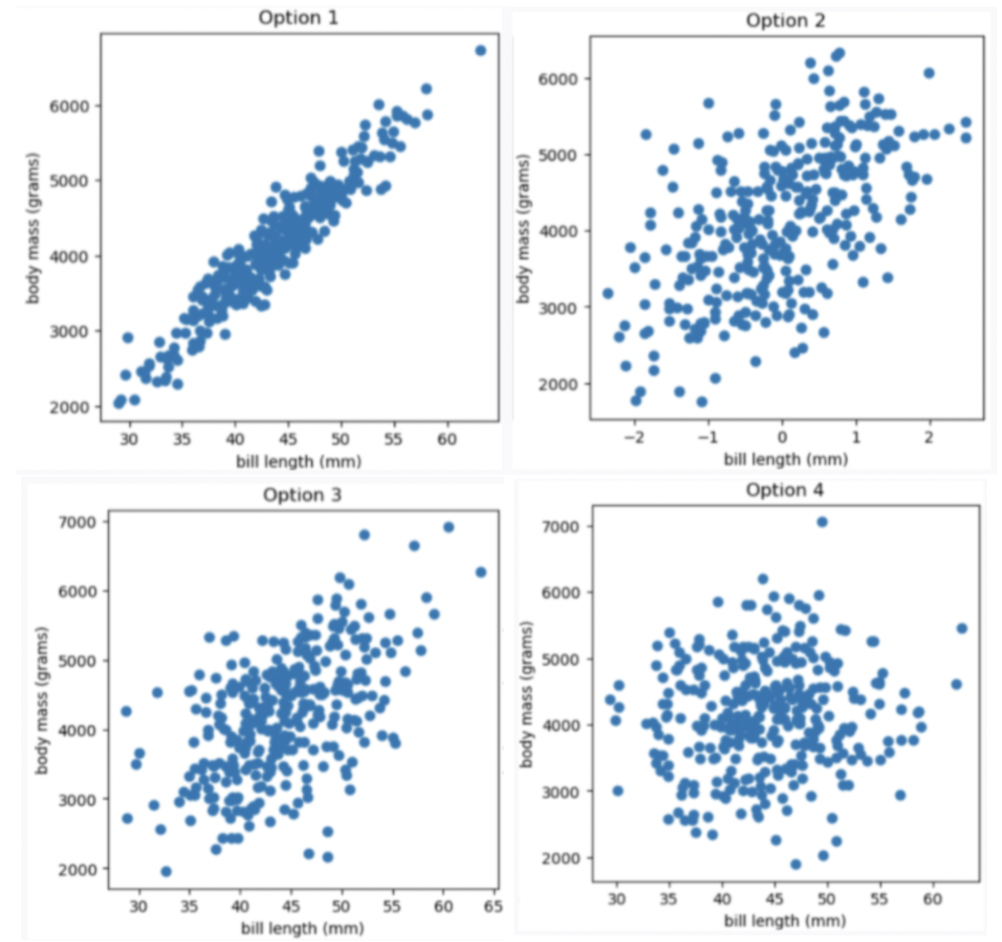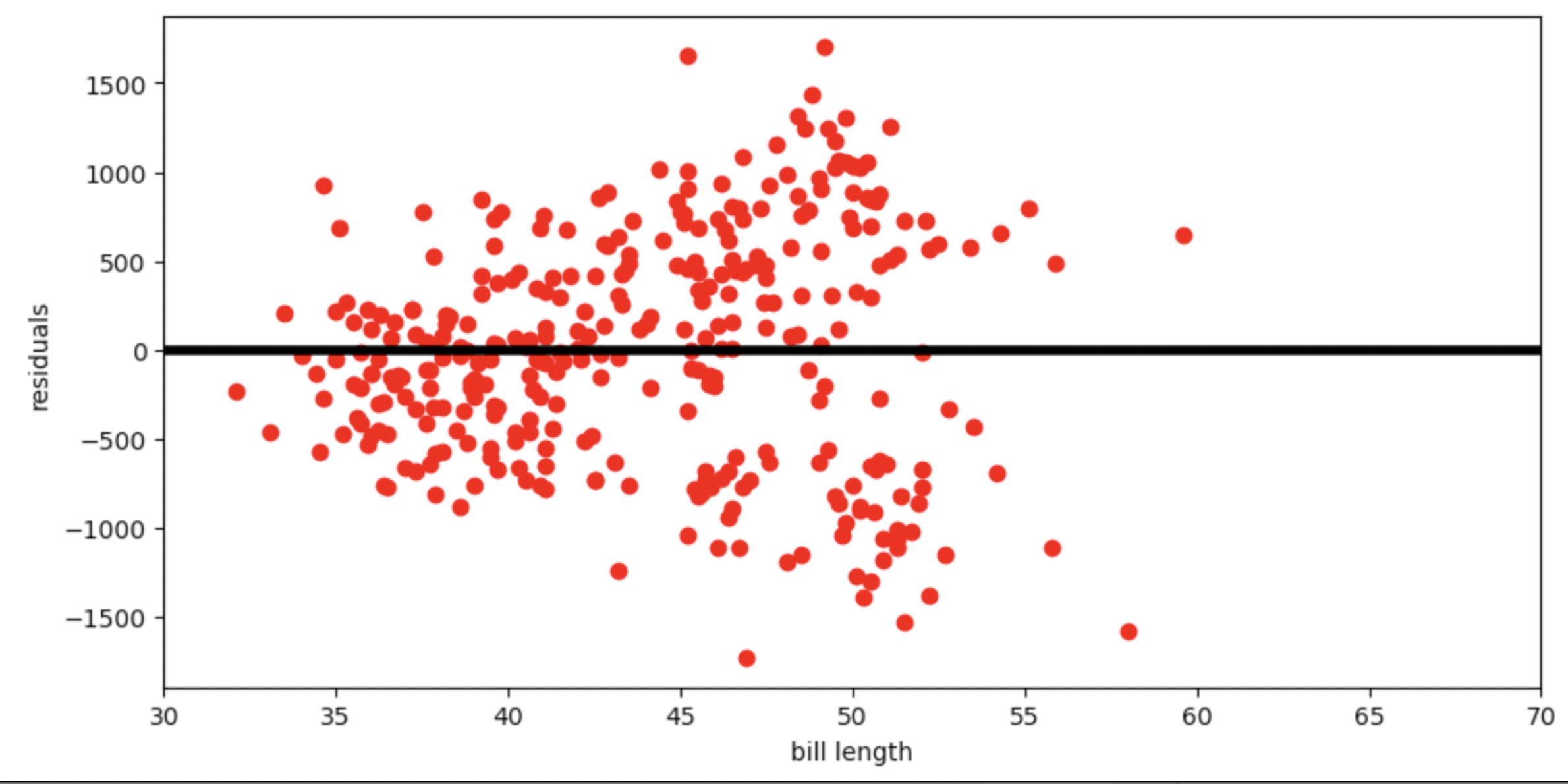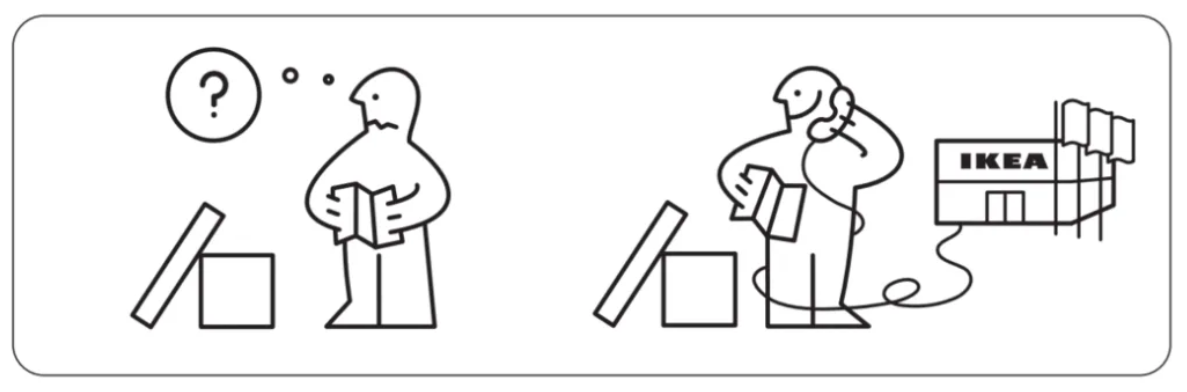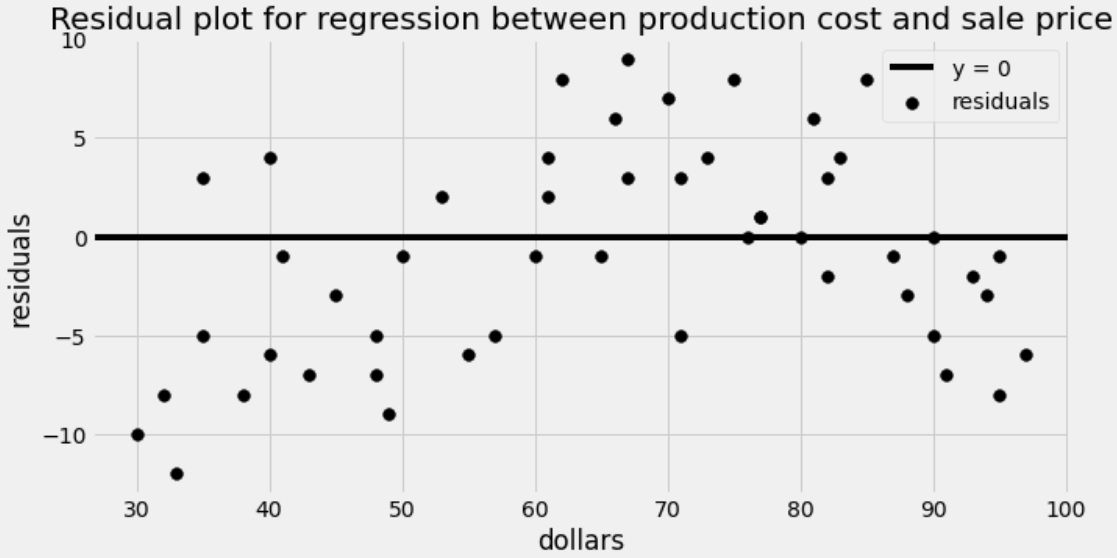# Discussion 10: Regression

The problems in this worksheet are taken from past exams. Work on them on paper, since the exams you take in this course will also be on paper.

We encourage you to complete this worksheet in a live discussion section. Solutions will be made available after all discussion sections have concluded. You don’t need to submit your answers anywhere.

Note: We do not plan to cover all problems here in the live discussion section; the problems we don’t cover can be used for extra practice.

## Problem 1

Let’s study the relationship between a penguin’s bill length (in millimeters) and mass (in grams). Suppose we’re given that

• bill length and body mass have a correlation coefficient of 0.55
• the average bill length is 44 mm and the standard deviation of bill lengths is 6 mm
• the average body mass is 4200 grams and the standard deviation of body mass is 840 grams

### Problem 1.1

Which of the four scatter plots below describe the relationship between bill length and body mass, based on the information provided in the question?• Option 1

• Option 2

• Option 3

• Option 4

Given the correlation coefficient is 0.55, bill length and body mass has a moderate positive correlation. We eliminate Option 1 (strong correlation) and Option 4 (weak correlation).

Given the average bill length is 44 mm, we expect our x-axis to have 44 at the middle, so we eliminate Option 2

##### Difficulty: ⭐️

The average score on this problem was 91%.

### Problem 1.2

Suppose we want to find the regression line that uses bill length, x, to predict body mass, y. The line is of the form y = mx +\ b. What are m and b?

What is m? Give your answer as a number without any units, rounded to three decimal places.

What is b? Give your answer as a number without units, rounded to three decimal places.

Answer: m = 77, b = 812

m = r \cdot \frac{\text{SD of }y }{\text{SD of }x} = 0.55 \cdot \frac{840}{6} = 77 b = \text{mean of }y - m \cdot \text{mean of }x = 4200-77 \cdot 44 = 812

##### Difficulty: ⭐️

The average score on this problem was 92%.

### Problem 1.3

What is the predicted body mass (in grams) of a penguin whose bill length is 44 mm? Give your answer as a number without any units, rounded to three decimal places.

y = mx\ +\ b = 77 \cdot 44 + 812 = 3388 +812 = 4200

##### Difficulty: ⭐️

The average score on this problem was 95%.

### Problem 1.4

A particular penguin had a predicted body mass of 6800 grams. What is that penguin’s bill length (in mm)? Give your answer as a number without any units, rounded to three decimal places.

In this question, we want to compute x value given y value y = mx\ +\ b y - b = mx \frac{y - b}{m} = x\ \ \text{(m is nonzero)} x = \frac{y - b}{m} = \frac{6800 - 812}{77} = \frac{5988}{77} \approx 77.766

##### Difficulty: ⭐️⭐️

The average score on this problem was 88%.

### Problem 1.5

Below is the residual plot for our regression line.Which of the following is a valid conclusion that we can draw solely from the residual plot above?

• For this dataset, there is another line with a lower root mean squared error

• The root mean squared error of the regression line is 0

• The accuracy of the regression line’s predictions depends on bill length

• The relationship between bill length and body mass is likely non-linear

• None of the above

Answer: The accuracy of the regression line’s predictions depends on bill length

The vertical spread in this residual plot is uneven, which implies that the regression line’s predictions aren’t equally accurate for all inputs. This doesn’t necessarily mean that fitting a nonlinear curve would be better. It just impacts how we interpret the regression line’s predictions.

##### Difficulty: ⭐️⭐️⭐️⭐️

The average score on this problem was 40%.

## Problem 2

IKEA is a Swedish furniture company that designs and sells ready-to-assemble furniture and other home furnishings. IKEA has locations worldwide, including one in San Diego. IKEA is known for its cheap prices, modern designs, huge showrooms, and wordless instruction manuals. They also sell really good Swedish meatballs in their cafe!Suppose the price of an IKEA product and the cost to have it assembled are linearly associated with a correlation of 0.8. Product prices have a mean of 140 dollars and a standard deviation of 40 dollars. Assembly costs have a mean of 80 dollars and a standard deviation of 10 dollars. We want to predict the assembly cost of a product based on its price using linear regression.

### Problem 2.1

The NORDMELA 4-drawer dresser sells for 200 dollars. How much do we predict its assembly cost to be?

We first use the formulas for the slope, m, and intercept, b, of the regression line to find the equation. For our application, x is the price and y is the assembly cost since we want to predict the assembly cost based on price.

\begin{aligned} m &= r*\frac{\text{SD of }y}{\text{SD of }x} \\ &= 0.8*\frac{10}{40} \\ &= 0.2\\ b &= \text{mean of }y - m*\text{mean of }x \\ &= 80 - 0.2*140 \\ &= 80 - 28 \\ &= 52 \end{aligned}

Now we know the formula of the regression line and we simply plug in x=200 to find the associated y value.

\begin{aligned} y &= mx+b \\ y &= 0.2x+52 \\ &= 0.2*200+52 \\ &= 92 \end{aligned}

##### Difficulty: ⭐️⭐️

The average score on this problem was 76%.

### Problem 2.2

The IDANÄS wardrobe sells for 80 dollars more than the KLIPPAN loveseat, so we expect the IDANÄS wardrobe will have a greater assembly cost than the KLIPPAN loveseat. How much do we predict the difference in assembly costs to be?

The slope of a line describes the change in y for each change of 1 in x. The difference in x values for these two products is 80, so the difference in y values is m*80 = 0.2*80 = 16 dollars.

An equivalent way to state this is:

\begin{aligned} m &= \frac{\text{ rise, or change in } y}{\text{ run, or change in } x} \\ 0.2 &= \frac{\text{ rise, or change in } y}{80} \\ 0.2*80 &= \text{ rise, or change in } y \\ 16 &= \text{ rise, or change in } y \end{aligned}

##### Difficulty: ⭐️⭐️⭐️

The average score on this problem was 65%.

### Problem 2.3

If we create a 95% prediction interval for the assembly cost of a 100 dollar product and another 95% prediction interval for the assembly cost of a 120 dollar product, which prediction interval will be wider?

• The one for the 100 dollar product.

• The one for the 120 dollar product.

Answer: The one for the 100 dollar product.

Prediction intervals get wider the further we get from the point (\text{mean of } x, \text{mean of } y) since all regression lines must go through this point. Since the average product price is 140 dollars, the prediction interval will be wider for the 100 dollar product, since it’s the further of 100 and 120 from 140.

##### Difficulty: ⭐️⭐️⭐️⭐️

The average score on this problem was 45%.

## Problem 3

For each IKEA desk, we know the cost of producing the desk, in dollars, and the current sale price of the desk, in dollars. We want to predict sale price based on production cost using linear regression.

### Problem 3.1

For this scenario, which of the following most likely describes the slope of the regression line when both variables are measured in dollars?

• less than 0

• between 0 and 1, exclusive

• more than 1

• none of the above (exactly equal to 0 or 1)

The slope of a line represents the change in y for each change of 1 in x. Therefore, the slope of the regression line is the amount we’d predict the sale price to increase when the production cost of an item increases by one dollar. In other words, it’s the sale price per dollar of production cost. This is almost certainly more than 1, otherwise the company would not make a profit. We’d expect that for any company, the sale price of an item should exceed the production cost, meaning the slope of the regression line has a value greater than one.

##### Difficulty: ⭐️⭐️⭐️

The average score on this problem was 72%.

### Problem 3.2

For this scenario, which of the following most likely describes the slope of the regression line when both variables are measured in standard units?

• less than 0

• between 0 and 1, exclusive

• more than 1

• none of the above (exactly equal to 0 or 1)

Answer: between 0 and 1, exclusive

When both variables are measured in standard units, the slope of the regression line is the correlation coefficient. Recall that correlation coefficients are always between -1 and 1, however, because it’s not realistic for production cost and sale price to be negatively correlated (as that would mean products sell for less if they cost more to produce) we can limit our choice of answer to values between 0 and 1. Because a coefficient of 0 would mean there is no correlation and 1 would mean perfect correlation (that is, plotting the data would create a line), these are unlikely occurrences leaving us with the answer being between 0 and 1, exclusive.

##### Difficulty: ⭐️⭐️

The average score on this problem was 86%.

### Problem 3.3

The residual plot for this regression is shown below.What is being represented on the horizontal axis of the residual plot?

• actual production cost

• actual sale price

• predicted production cost

• predicted sale price

Residual plots show x on the horizontal axis and the residuals, or differences between actual y values and predicted y values, on the vertical axis. Therefore, the horizontal axis here shows the production cost. Note that we are not predicting production costs at all, so production cost means the actual cost to produce a product.

##### Difficulty: ⭐️⭐️⭐️⭐️

The average score on this problem was 43%.

### Problem 3.4

Which of the following is a correct conclusion based on this residual plot? Select all that apply.

• The correlation between production cost and sale price is weak.

• It would be better to fit a nonlinear curve.

• Our predictions will be more accurate for some inputs than others.

• We don’t have enough data to do regression.

• The regression line is not the best-fitting line for this data set.

• The data set is not representative of the population.

Answer: It would be better to fit a nonlinear curve.

Let’s go through each answer choice.

• The correlation between production cost and sale price could be very strong. After all, we are able to predict the sale price within ten dollars almost all the time, since residuals are almost all between -10 and 10.

• It would be better to fit a nonlinear curve because the residuals show a pattern. Reading from left to right, they go from mostly negative to mostly positive to mostly negative again. This suggests that a nonlinear curve might be a better fit for our data.

• Our predictions are typically within ten dollars of the actual sale price, and this is consistent throughout. We see this on the residual plot by a fairly even vertical spread of dots as we scan from left to right. This data is not heteroscedastic.

• We can do regression on a dataset of any size, even a very small data set. Further, this dataset is decently large, since there are a good number of points in the residual plot.

• The regression line is always the best-fitting line for any dataset. There may be other curves that are better fits than lines, but when we restrict to lines, the best of the bunch is the regression line.

• We have no way of knowing how representative our data set is of the population. This is not something we can discern from a residual plot because such a plot contains no information about the population from which the data was drawn.

##### Difficulty: ⭐️⭐️⭐️

The average score on this problem was 61%.

## Problem 4

### Problem 4.1

True or False: The slope of the regression line, when both variables are measured in standard units, is never more than 1.

Standard units standardize the data into z scores. When converting to Z scores the scale of both the dependent and independent variables are the same, and consequently, the slope can at most increase by 1. Alternatively, according to the reference sheet, the slope of the regression line, when both variables are measured in standard units, is also equal to the correlation coefficient. And by definition, the correlation coefficient can never be greater than 1 (since you can’t have more than a ‘perfect’ correlation).

##### Difficulty: ⭐️

The average score on this problem was 93%.

### Problem 4.2

True or False: The slope of the regression line, when both variables are measured in original units, is never more than 1.

Original units refers to units as they are. Clearly, regression slopes can be greater than 1 (for example if for every change in 1 unit of x corresponds to a change in 20 units of y the slope will be 20).

##### Difficulty: ⭐️

The average score on this problem was 96%.

## Problem 5

### Problem 5.1

Are nonfiction books longer than fiction books?

• hypothesis testing

• permutation (A/B) testing

• Central Limit Theorem

• regression

The question Are nonfiction books longer than fiction books? is investigating the difference between two underlying populations (nonfiction books and fiction books). A permutation test is the best data science tool when investigating differences between two underlying distributions.

##### Difficulty: ⭐️

The average score on this problem was 90%.

### Problem 5.2

Do people have more friends as they get older?

• hypothesis testing

• permutation (A/B) testing

• Central Limit Theorem

• regression

The question at hand is investigating two continuous variables (time and number of friends). Regression is the best data science tool as it is dealing with two continuous variables and we can understand correlations between time and the number of friends.

##### Difficulty: ⭐️

The average score on this problem was 90%.

### Problem 5.3

Does an ice cream shop sell more chocolate or vanilla ice cream cones?

• hypothesis testing

• permutation (A/B) testing

• Central Limit Theorem

• regression